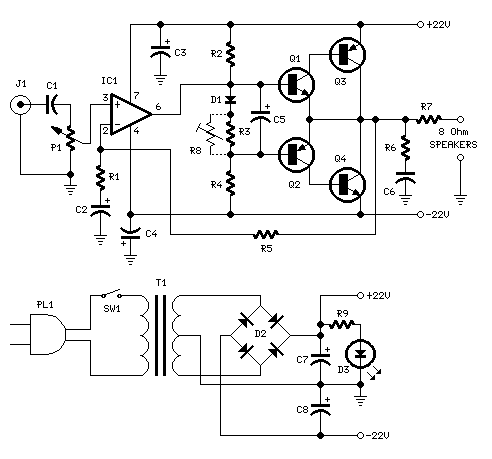# 18W的音频放大器电路（TLE2141C/TIP42A/TIP41A）P1 = 22K 电位器
R1 = 1K 1/4W电阻
1/4W电阻R2 = 4K7
R3 = 100R 1/4W电阻
R4 = 4K7 1/4W电阻
R5 = 82K 1/4W电阻
R6 = 10R 1/2W电阻
R7 = R22 4W电阻（绕线）
R8 = 1K 1/2W金属陶瓷微调（可选）
C1 = 470nF的63V涤纶电容器
C2，C5 = 100uF的3V珠钽电容器
C3，C4 = 470uF的25V电​​解电容器
C6 = 100nF的电容63V涤纶
D1 = 1N4148 75V 150mA的二极管
IC1 = TLE2141C低噪音，高电压，高转换率的运算放大器
Q1 = BC182 50V 100MA NPN晶体管
Q2 = BC212 50V 100MA PNP晶体管
Q3 = TIP42A 60V 6A PNP晶体管
Q4 = TIP41A 6A 60V NPN晶体管
J1的RCA音频输入插座

R9 = 2K2 1/4W电阻
C7，C8 = 4700UF 25V电解电容器
D2 100V 4A二极管桥
D35毫米。 红色LED
T1 220V，15 + 15V，50VA电源变压器
PL1电源插头
SW1单刀单掷（SPST）电源开关

Q3和Q4必须安装散热片。
D1必须接触散热片。